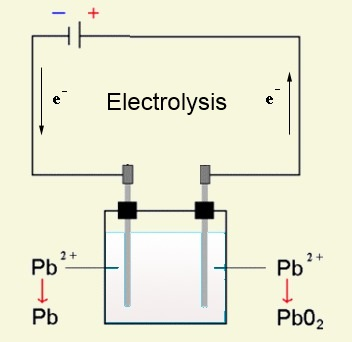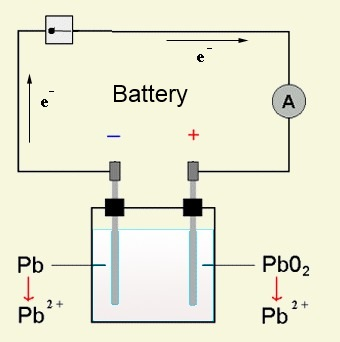Search:

# Accumulators

## Definition

Accumulators are rechargeable batteries by électrolysis.

The accumulator consists of lead electrodes immersed in sulfuric acid. The sulfuric acids attacks the lead plates superficially covering them with a layer of lead sulfate: $2H^+$ $+$ $SO_4^{2-}$ $+$ $Pb$ $\longrightarrow$ $Pb^{2+}SO_4^{2-}$ $+$ $H_2$ The lead sulfate layer est very thin and impermeable. In the following interpretation we shall consider the $Pb^{2+}$ ion alone which is contained in the lead sulfate layer.

Charge     =     ElectrolysisAt the cathode: $Pb^{2+}$ $+$ $2e^-$ $\longrightarrow$ $Pb$ ( deposit on the electrode ) At the anode: $Pb^{2+}$ $+$ $2H_2O$ $-$ $2e^-$ $\longrightarrow$ $4H^+$ $+$ $PbO_2$ (deposit on the electrode )

Discharge     =     BatteryOn the side of the reductant $Pb$: $Pb$ $-$ $2e^-$ $\longrightarrow$ $Pb^{2+}$ ($\ominus$ pole) On the side of the oxidant $PbO_2$: $PbO_2$ $+$ $4H^+$ $+$ $2e^-$ $\longrightarrow$ $Pb^{2+}$ $+$ $2H_2O$ ($\oplus$ pole)